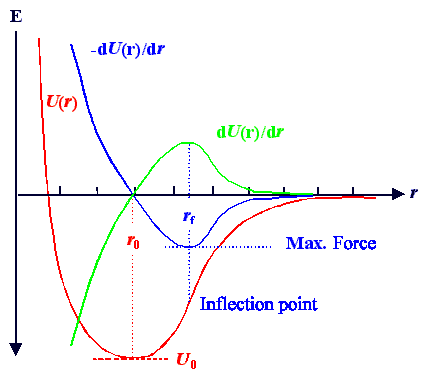### 2.4.3 Maximale Bruchspannung

Definition

Dieser Modul ist in Englisch, da er auch in anderen Hyperskripten gebraucht wird.
Some variables are not written in italics for ease of writingLets see what happens if we pull a crystal apart by applying sufficient force (this is always possible, remember the first law of material science :-)The force needed to move an atom off the equilibrium position at r0 in the potential U(r) is given by dU/dr (the negative sign used in the definition of a potential is always the restoring force, i.e. the force that drives a particle in the direction towards the potential minimum).A schematic drawing of a typical potential well together with dU/dr is shown below.We notice several features:1. At the potential minimum at r0 , the force must be zero.
2. If the force curve is rather linear in going through r0, the potential around r0 is rather quadratic, we have a small thermal expansion and the vibration will be a harmonic oscillation.
3. The force goes through a maximum at r = rf. This means that dF/dr |rf = 0 and thus d2U/dr 2 |rf = 0. rf thus is the position of the inflection point of the potential curve.What does the force maximum mean? Simple:Moving the atom to the point rf needs the ultimate amount of force that we need in order to tear the atoms apart. If we want to move it even further away from its equilibrium position, the force needed after reaching rf can decrease again.So if we can apply the force Ff = F(rf), we will fracture the crystal for sure. Ff thus defines the ultimate fracture strength of the material.Easy, but a bit misleading!If you pull at a given material with some external force Fext, you apply some mechanical stress and this translates into a defined force per bond.Now you double the external force. Does the force per bond double, too? Of course you are tempted to say, but that is not always true.Materials that can undergo plastic deformation (all metals and many others) have a tricky mechanism which allows them to reduce the internal stress by "yielding", by deforming plastically.The strain going with any stress then can be much larger than what we are going to calculate. You simply never built up enough internal stress to break the material, it first gets longer and longer (and thinner) before it eventually falls apart.

CalculationThe calculation of Ff  is straight forward.We have Ff = dU/dr |rf  and d2U/dr2 = 0|rf.So first we calculate rf. We already have the second derivative from sub chapter 2.4.2, it was
d2U
=  U''  =  n(n + 1)
r 2
· A · r –n   +   m(m + 1)
r 2
· B · r -m
dr 2Substituting A and B by r0 and U0 (taken from the solution to exercise 2.4-1) yields
A  =  U0 · r0n ·    m
m – n

B  =  U0 · r0m ·    n
n – mIn total we obtain
d2U
|rf  =  0  =  – n(n + 1) · rf –n
·  m · U0 · r0n
+   m · (m + 1) · rf –m
·  n · U0 · r0m
dr 2 rf2 m – n rf2 n – mThat looks worse than it is. The denominators, the mn products, and theU0 disappear after some juggling, we have
 [(n + 1) · r0n · rf – n] + [(m + 1) · r0m  · rf – m]  =  0which finally gives us
 rf  =  r0 n + 1 æ ç è ö ÷ øA not too involved formula, but not overly helpful either - we have a strong dependence on the somewhat fishy parameters n and m. Lets see what we can deduce.Lets look at an ionic bond where we have n = 1 and m = 8...12. This gives
rf = r0(2/9 ... 2/13)– 1/7... –1/11 = (1,306...1,185)r0,
or a maximum strain until fracture ofef = (rf - r0)/r0 = (rf /r0 – 1) = (0,306...0,185)
or an ultimate fracture strain of 18% - 30%.Calculating the force needed for ultimate fracture now is possible, but not extremely useful. For a first approximation we can just calculate the ultimate fracture stress sf  by using Youngs modulus E via sf = E · ef.In chapter 2.4.1 we obtained E = n · m ·U0 /r03, and that gives us
 sf  = n · m · U0 n + 1 1/(n – m)   r03 æ ç è æ ç è ö ÷ ø ö ÷ øThat looks like a complicated formula, but all it says is that the ultimate fracture stress is in the order of 10% ... 30 % of Youngs modulus itself. We will encounter this statement later again, but from a quite different consideration.The calculation of Youngs modulus and the thermal expansion coefficient were quite satisfactory in comparison to actual values. How good is estimate of the ultimate fracture strength based on bonding potentials?Not very good, as it turns out. In fact, observed fracture toughness is often quite smaller (like two orders of magnitude) and often materials start to deform heavily, albeit plastically, but leading to eventual fracture, at much lower stress levels, but much larger strain.The reason for that is that we did not take into account defects in the crystal lattice. In contrast to Youngs modulus, the melting point, and the thermal expansion coefficient: Fracture toughness is a defect sensitive property.We must therefore give some thought to crystal lattice defects soon.

© H. Föll (MaWi 1 Skript)